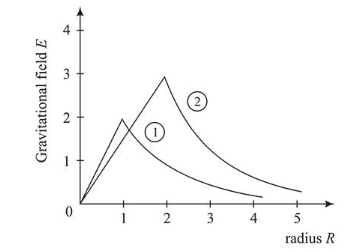# Consider two solid spheres of radiiQuestion:

Consider two solid spheres of radii $R_{1}=1 m, R_{2}=2 m$ and masses $M_{1}$ and $M_{2}$, respectively. The gravitational field due to sphere (1) and (2) are shown. The value of $\frac{m_{1}}{m_{2}}$ is:1. (1) $\frac{2}{3}$

2. (2) $\frac{1}{6}$

3. (3) $\frac{1}{2}$

4. (4) $\frac{1}{3}$

Correct Option: , 2

Solution:

(2) Gravitation field at the surface

$E=\frac{G m}{r^{2}}$

$\therefore E_{1}=\frac{G m_{1}}{r_{1}^{2}}$ and $E_{2}=\frac{G m_{2}}{r_{2}^{2}}$

From the diagram given in question,

$\frac{E_{1}}{E_{2}}=\frac{2}{3}\left(r_{1}=1 \mathrm{~m}, R_{2}=2 m\right.$ given $)$

$\therefore \frac{E_{1}}{E_{2}}=\left(\frac{r_{2}}{r_{1}}\right)^{2}\left(\frac{m_{1}}{m_{2}}\right) \Rightarrow \frac{2}{3}=\left(\frac{2}{1}\right)^{2}\left(\frac{m_{1}}{m_{2}}\right)$

$\Rightarrow\left(\frac{m_{1}}{m_{2}}\right)=\frac{1}{6}$